### Home > A2C > Chapter Ch13 > Lesson 13.2.2 > Problem13-112

13-112.
1. Without using a calculator, solve each equation below in the domain 0 ≤ x ≤ 2π. Homework Help ✎

1. sin(x) = −1

2. 2cos(x) −1 = 0

3. tan(x) = 1

4. 2sin(x) = 4sin(x) + 1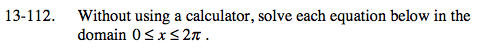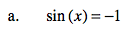x = π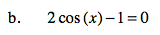$\text{cos}(x)=\frac{1}{2}$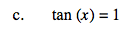$x=\frac{\pi}{4},\,\frac{5\pi}{4}$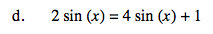$\text{sin}(x)=-\frac{1}{2}$